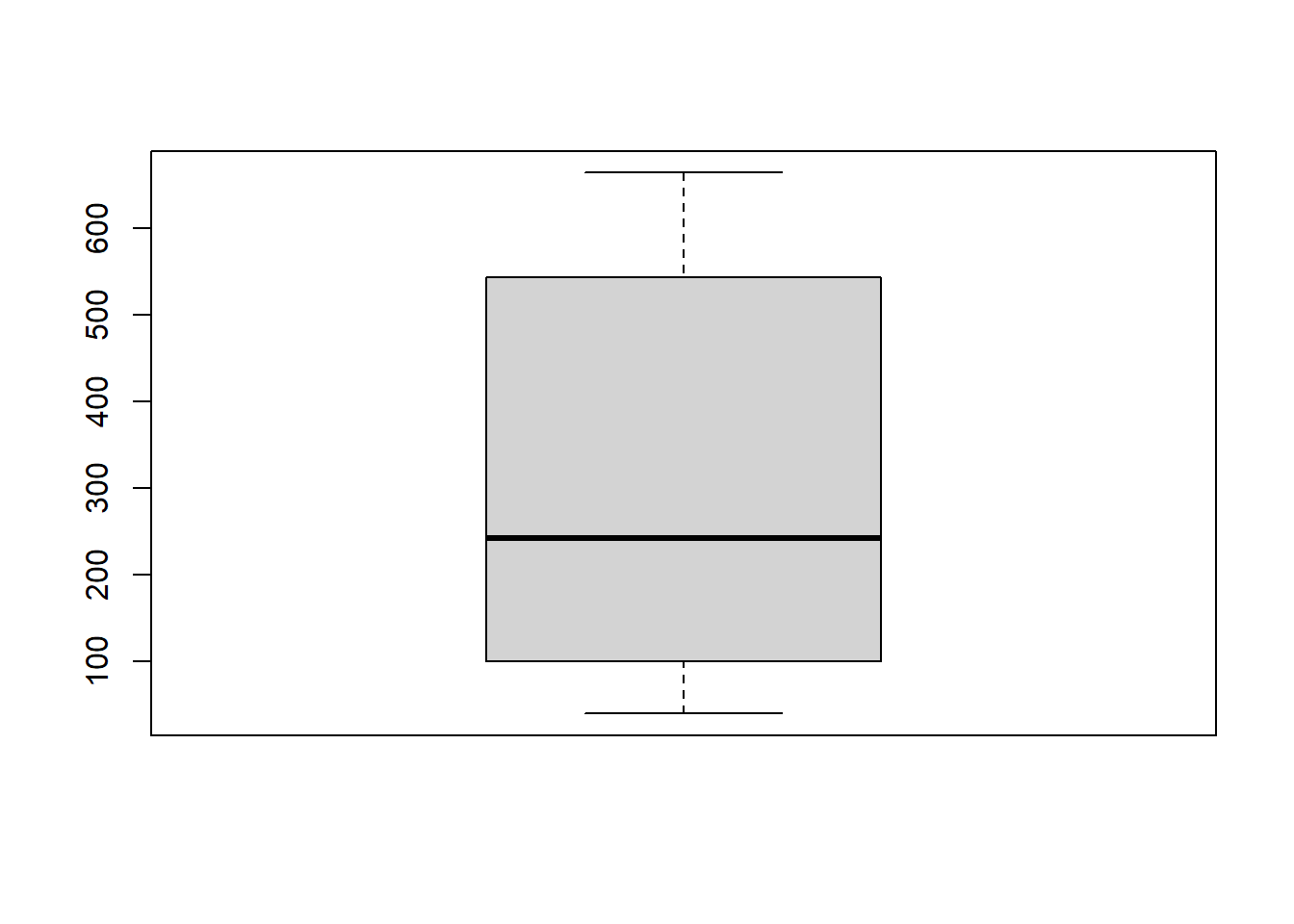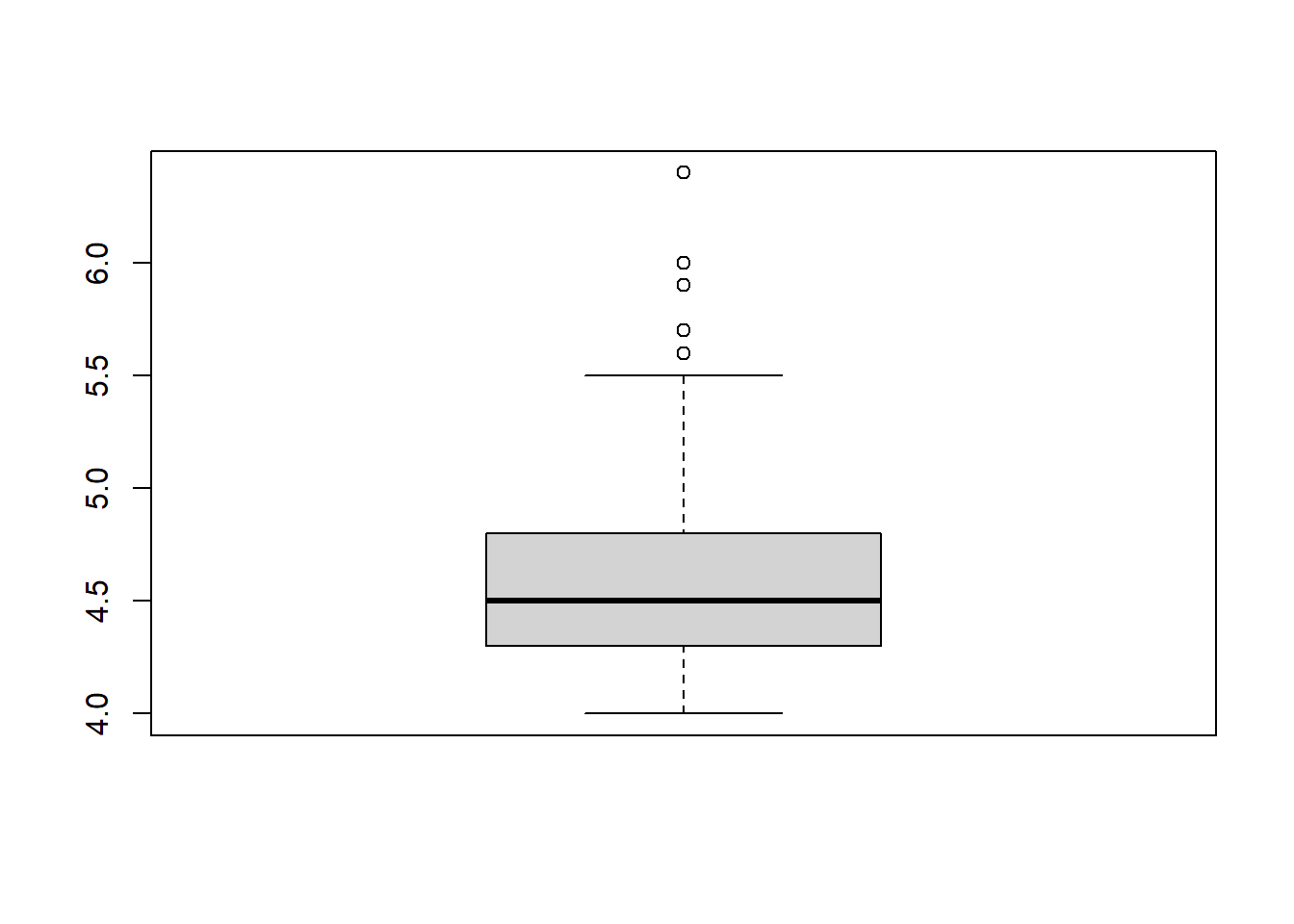``````# 1) Data Exploration: This should include summary statistics, means, medians, quartiles, or any
# other relevant information about the data set.

``````## New names:
## * `` -> ...1``````
``````View(quakes)

summary(quakes\$mag)``````
``````##    Min. 1st Qu.  Median    Mean 3rd Qu.    Max.
##    4.00    4.30    4.60    4.62    4.90    6.40``````
``summary(quakes\$stations)``
``````##    Min. 1st Qu.  Median    Mean 3rd Qu.    Max.
##   10.00   18.00   27.00   33.42   42.00  132.00``````
``summary(quakes\$depth)``
``````##    Min. 1st Qu.  Median    Mean 3rd Qu.    Max.
##    40.0    99.0   247.0   311.4   543.0   680.0``````
``var(quakes\$mag)``
``##  0.1622261``
``sd(quakes\$mag)``
``##  0.402773``
``````# Data wrangling: Please perform some basic transformations. They will need to make sense but
# could include column renaming, creating a subset of the data, replacing values, or creating new
# columns with derived data (for example - if it makes sense you could sum two columns together)

#     take a sample range of the data
#     rename abbreviations to longitude, latitude, magnitude
#     Drop numbered column

samples <- quakes[c(100:250, 700:1000),c(2:6)]
print(samples)``````
``````## # A tibble: 452 x 5
##      lat  long depth   mag stations
##    <dbl> <dbl> <dbl> <dbl>    <dbl>
##  1 -24.6  180.   484   4.7       33
##  2 -17.0  186.   108   4.1       12
##  3 -26.2  178.   583   4.6       25
##  4 -21.9  180.   608   4.7       30
##  5 -33    182.    72   4.7       22
##  6 -21.3  181.   636   4.6       29
##  7 -19.4  184.   293   4.2       15
##  8 -34.9  181.    42   4.4       25
##  9 -20.2  169.   100   4.6       22
## 10 -22.6  186.    42   5.7       76
## # ... with 442 more rows``````
``````names(samples) <- c("Latitude", "Longitude", "Depth", "Magnitude", "Station")
print(samples)``````
``````## # A tibble: 452 x 5
##    Latitude Longitude Depth Magnitude Station
##       <dbl>     <dbl> <dbl>     <dbl>   <dbl>
##  1    -24.6      180.   484       4.7      33
##  2    -17.0      186.   108       4.1      12
##  3    -26.2      178.   583       4.6      25
##  4    -21.9      180.   608       4.7      30
##  5    -33        182.    72       4.7      22
##  6    -21.3      181.   636       4.6      29
##  7    -19.4      184.   293       4.2      15
##  8    -34.9      181.    42       4.4      25
##  9    -20.2      169.   100       4.6      22
## 10    -22.6      186.    42       5.7      76
## # ... with 442 more rows``````
``summary(samples)``
``````##     Latitude        Longitude         Depth         Magnitude
##  Min.   :-38.59   Min.   :165.8   Min.   : 40.0   Min.   :4.000
##  1st Qu.:-23.47   1st Qu.:179.7   1st Qu.:100.0   1st Qu.:4.300
##  Median :-20.52   Median :181.5   Median :242.0   Median :4.500
##  Mean   :-20.68   Mean   :179.7   Mean   :310.2   Mean   :4.596
##  3rd Qu.:-17.82   3rd Qu.:183.5   3rd Qu.:543.2   3rd Qu.:4.800
##  Max.   :-10.80   Max.   :188.1   Max.   :664.0   Max.   :6.400
##     Station
##  Min.   : 10.00
##  1st Qu.: 18.00
##  Median : 27.00
##  Mean   : 33.68
##  3rd Qu.: 42.00
##  Max.   :132.00``````
``sum(samples\$Station)``
``##  15223``
``````# Graphics: Please make sure to display at least one scatter plot, box plot and histogram. Don't
# be limited to this. Please explore the many other options in R packages such as ggplot2.

boxplot(samples\$Depth)````````boxplot(samples\$Magnitude)````hist(samples\$Depth)``